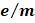# Which of the following is true? a) The outer electronic configuration of the ground state chromium atom isb) Gamma rays are electromagnetic radiations of wavelengths ofcm tocm c) The energy of the electron in theorbital is less than that in theorbital of a hydrogen atom d) The electron density in the-plane inorbital is zero

## Question ID - 101879 :- Which of the following is true? a) The outer electronic configuration of the ground state chromium atom isb) Gamma rays are electromagnetic radiations of wavelengths ofcm tocm c) The energy of the electron in theorbital is less than that in theorbital of a hydrogen atom d) The electron density in the-plane inorbital is zero

3537

(c)

1. False. The configuration is2. False. The wavelength of gamma rays is of the order ofm

3. True. In hydrogen atom, the energy of an electron depends only on the principal quantum number of the orbital which it occupies

False. In-plane, there is no electron density if an electron occupiesorbital

Next Question :
 The increasing order (lowest first) for the values of(charge/mass) for electron, proton, neutron, and alpha particleis a)b)c)d)